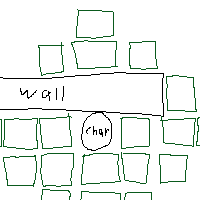# Tile Movement system can't account for obstacles

I have a grid-based or tile-based movement system that can move a selected character up to their movement range away from their current tile. This works perfectly fine for flat surfaces where there are no obstructions, but as soon as I add obstacles to the map, the characters can completely ignore them and phase right through without account for their movement range (e.g. rather than needing to walk past a wall, they can phase right through).

What I want is a way to calculate which tiles they can move to with respect to any obstacles in their path. I have no idea how to achieve this, and can’t find any resources. Here’s a dumbed down rblx file since I know this is kind of complicated to solve without getting hands on

Baseplate.rbxl (206.4 KB)

This function is from a script that is a localscript in StarterPlayerScripts that controls everything related to mouse controls. The function checks every block to see if it is within movement range, and if the tile is within movement range and not an obstacle, teammate, or enemy, the function marks the tile as being able to move to.

``````local function check(reset)
for i,v in pairs(Blocks:GetChildren()) do
v:SetAttribute("InRange", false)
end
for i,v in pairs(game.Workspace.CursorTiles:GetChildren()) do
v:Destroy()
end
SelectedX.Value = Selected.Value.CharacterStats.X.Value or script.CharacterUsed.Value.CharacterStats.X.Value
SelectedY.Value = Selected.Value.CharacterStats.Y.Value or script.CharacterUsed.Value.CharacterStats.Y.Value
SelectedMove.Value = Selected.Value.CharacterStats.MovementRange.Value or script.CharacterUsed.Value.CharacterStats.MovementRange.Value
for i,v in pairs(Blocks:GetChildren()) do
v:SetAttribute("InRange", false)
end
for i,v in pairs(Blocks:GetChildren()) do --Goes through all the blocks
tempX.Value = math.abs(v:GetAttribute("X") - SelectedX.Value)
tempY.Value = math.abs(v:GetAttribute("Y") - SelectedY.Value)
if tempX.Value + tempY.Value <= SelectedMove.Value and tempX.Value + tempY.Value ~= 0 and v:GetAttribute("Obstructed") == false and v:GetAttribute("HasEnemy") == false and v:GetAttribute("HasCharacter") == false and PlayerTurn.Value == true and Selected.Value.CharacterStats.AP.Value >= 1 and GlobalVariables.Planning.Value ~= true and Selected.Value.Parent ~= workspace.Enemies then

local newTile = ReplicatedStorage.MoveTile:Clone()
newTile.Parent = game.Workspace.CursorTiles
newTile.Position = v.Position + Vector3.new(0, 0.6, 0)
v:SetAttribute("InRange", true)

elseif tempX.Value + tempY.Value <= SelectedMove.Value and tempX.Value + tempY.Value ~= 0 and v:GetAttribute("Obstructed") == false and v:GetAttribute("HasEnemy") == false and v:GetAttribute("HasCharacter") == false and PlayerTurn.Value == true and GlobalVariables.Planning.Value ~= true and Selected.Value.CharacterStats.AP.Value <= 0 and Selected.Value.Parent ~= workspace.Enemies then
local newTile = ReplicatedStorage.NoAPMoveTile:Clone()
newTile.Parent = game.Workspace.CursorTiles
newTile.Position = v.Position + Vector3.new(0, 0.6, 0)
v:SetAttribute("InRange", false)
elseif tempX.Value + tempY.Value <= SelectedMove.Value and tempX.Value + tempY.Value ~= 0 and v:GetAttribute("Obstructed") == false and v:GetAttribute("HasCharacter") == false and PlayerTurn.Value == true and GlobalVariables.Planning.Value ~= true and Selected.Value.Parent == workspace.Enemies then
local newTile = ReplicatedStorage.EnemyMoveTile:Clone()
newTile.Parent = game.Workspace.CursorTiles
newTile.Position = v.Position + Vector3.new(0, 0.6, 0)
v:SetAttribute("InRange", false)
end
end
if reset == true then
for i,v in pairs(game.Workspace.CursorTiles:GetChildren()) do
v:Destroy()
end

for i,v in pairs(Blocks:GetChildren()) do --Goes through all the blocks
tempX.Value = math.abs(v:GetAttribute("X") - SelectedX.Value)
tempY.Value = math.abs(v:GetAttribute("Y") - SelectedY.Value)
if tempX.Value + tempY.Value <= SelectedMove.Value and tempX.Value + tempY.Value ~= 0 and v:GetAttribute("Obstructed") == false and v:GetAttribute("HasEnemy") == false and v:GetAttribute("HasCharacter") == false and PlayerTurn.Value == true and Selected.Value.CharacterStats.AP.Value >= 1 and GlobalVariables.Planning.Value ~= true and Selected.Value.Parent ~= workspace.Enemies then

local newTile = ReplicatedStorage.MoveTile:Clone()
newTile.Parent = game.Workspace.CursorTiles
newTile.Position = v.Position + Vector3.new(0, 0.6, 0)
v:SetAttribute("InRange", true)

elseif tempX.Value + tempY.Value <= SelectedMove.Value and tempX.Value + tempY.Value ~= 0 and v:GetAttribute("Obstructed") == false and v:GetAttribute("HasEnemy") == false and v:GetAttribute("HasCharacter") == false and PlayerTurn.Value == true and GlobalVariables.Planning.Value ~= true and Selected.Value.CharacterStats.AP.Value <= 0 and Selected.Value.Parent ~= workspace.Enemies then
local newTile = ReplicatedStorage.NoAPMoveTile:Clone()
newTile.Parent = game.Workspace.CursorTiles
newTile.Position = v.Position + Vector3.new(0, 0.6, 0)
v:SetAttribute("InRange", false)
elseif tempX.Value + tempY.Value <= SelectedMove.Value and tempX.Value + tempY.Value ~= 0 and v:GetAttribute("Obstructed") == false and v:GetAttribute("HasCharacter") == false and PlayerTurn.Value == true and GlobalVariables.Planning.Value ~= true and Selected.Value.Parent == workspace.Enemies then
local newTile = ReplicatedStorage.EnemyMoveTile:Clone()
newTile.Parent = game.Workspace.CursorTiles
newTile.Position = v.Position + Vector3.new(0, 0.6, 0)
v:SetAttribute("InRange", false)
end
end
if PlayerTurn.Value == false then
game.Workspace.GlobalVariables.Selected.Value = nil
script.SelectedX.Value = nil
script.SelectedY.Value = nil
script.tempX.Value = nil
script.tempY.Value = nil
end
script.BlockValue.Value = nil
script.SelectedMove.Value = nil
script.newHighlight.Value = nil
script.CharacterUsed.Value = nil
end
end
``````
1 Like

You can use OverlapParams to check if a tile is not obstructed (see Introducing OverlapParams - New Spatial Query API).

1 Like

I can already mark tiles as being obstacles so the characters can’t be on those tiles, but I’m not sure how to make them impassable so that you must walk around them rather than through them

here’s how my current system works:here’s how I want it to work: